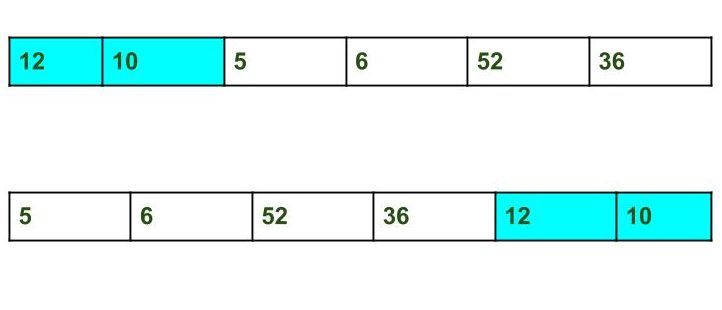Open in App
Not now

# Java Program to Split the array and add the first part to the end | Set 2

• Last Updated : 24 Jan, 2022

Given an array and split it from a specified position, and move the first part of array add to the end.Examples:

```Input : arr[] = {12, 10, 5, 6, 52, 36}
k = 2
Output : arr[] = {5, 6, 52, 36, 12, 10}
Explanation : Split from index 2 and first
part {12, 10} add to the end .

Input : arr[] = {3, 1, 2}
k = 1
Output : arr[] = {1, 2, 3}
Explanation : Split from index 1 and first

A O(n*k) solution is discussed here
This problem can be solved in O(n) time using the reversal algorithm discussed below,
1. Reverse array from 0 to n – 1 (where n is size of the array).
2. Reverse array from 0 to n – k – 1.
3. Reverse array from n – k to n – 1.

## Java

 `// Java program to Split the array and``// add the first part to the end``class` `Geeks``{``     ` `/* Function to reverse arr[] from index start to end*/``static` `void` `rvereseArray(``int` `arr[], ``int` `start, ``int` `end)``{``    ``while` `(start < end) {``        ``int` `temp = arr[start];``        ``arr[start] = arr[end];``        ``arr[end] = temp;``        ``start++;``        ``end--;``    ``}``}`` ` `// Function to print an array``static` `void` `printArray(``int` `arr[], ``int` `size)``{``    ``for` `(``int` `i = ``0``; i < size; i++)``        ``System.out.print(arr[i] +``" "``);``}`` ` `/* Function to left rotate arr[] of size n by k */``static` `void` `splitArr(``int` `arr[], ``int` `k, ``int` `n)``{``    ``rvereseArray(arr, ``0``, n - ``1``);``    ``rvereseArray(arr, ``0``, n - k - ``1``);``    ``rvereseArray(arr, n - k, n - ``1``);``}`` ` `/* Driver program to test above functions */``public` `static` `void` `main(String args[])``{``    ``int` `arr[] = { ``12``, ``10``, ``5``, ``6``, ``52``, ``36` `};``    ``int` `n = arr.length;``    ``int` `k = ``2``;`` ` `    ``// Function calling``    ``splitArr(arr, k, n);``    ``printArray(arr, n);`` ` `}`` ` `}`` ` `// This code is contributed by ankita_saini.`

Output:

`5 6 52 36 12 10 `

Please refer complete article on Split the array and add the first part to the end | Set 2 for more details!

My Personal Notes arrow_drop_up